Mathematical and Physical Journal
for High Schools
Issued by the MATFUND Foundation
 Already signed up? New to KöMaL?# Solutions for problems "B" in September, 2001

In this page only the sketch of the solutions are published; in some cases only the final results. To achieve the maximum score in the competition more detailed solutions needed.

B. 3472. How many time instants are there between 6 a.m. and 6 p.m. when it is not possible to tell the time if we cannot distinguish the long and short hands of the clock?  (3 points)

Kvant

Solution. Suppose the positions of the two hands are the same at b minutes past a o'clock as at d minutes past c o'clock, but with the short and long hands interchanged. Here 0$\displaystyle le$a,c$\displaystyle le$11 are integers, and 0$\displaystyle le$b,d<60 are real numbers. (For simplicity, we say 0 instead of 12 o'clock.) Thus 5a+b/12=d and 5c+d/12=a. Hence b and d can be expressed in terms of a and c as follows:

$\displaystyle b={60a+720c\over143},\quad d={60c+720a\over143}.$

If a=c, then b=d is also true, and in the case of 0$\displaystyle le$a,c$\displaystyle le$11, a$\displaystyle ne$c, the values of both b and d are between 0 and 60. For every integer 0$\displaystyle le$a$\displaystyle le$11 there are exactly 11 such time instants between a o'clock and a+1 o'clock. Thus there are 12.11=132 such time instants between 6 a.m. and 6 p.m.

B. 3473. The area of parallelogram ABCD is 2 units. The line parallel to AD intersects the boundary of the parallelogram at P and R, and the line parallel to AB intersects it at S and Q, as shown in the figure. What is the total area of the triangles AQR, BSR, DPQ and CSP?  (3 points)Solution. If AP=xAB and AS=yAD then the area of triangle ABQ is y,  the area of triangle ARD is x, and that of triangle CRQ is (1-x)(1-y).  Hence the area of triangle AQR is 2-x-y-(1-x)(1-y). With a similar reasoning, the areas of triangles BSR, DPQ and CSP are 2-(1-x)-y-x(1-y), 2-x-(1-y)-(1-x)y and 2-(1-x)-(1-y)-xy, respectively. By adding up the areas, we get

8-2(x+(1-x))-2(y+(1-y))-(x+(1-x))(y+1-y))=3.

for the total area.

B. 3474. What is the seventy-third digit from the back in the number $\displaystyle (\underbrace{111\dots1}_{112})^2$?  (4 points)

Solution. The seventy-third digit from the right in $\displaystyle {\underbrace{111\dots1}_{112}}^2$ equals the seventy-third digit from the right in

$\displaystyle A={\underbrace{111\dots1}_{73}}+{\underbrace{111\dots1}_{72}}0+{\underbrace{111\dots1}_{71}}00+\ldots11{\underbrace{000\dots0}_{71}}+1{\underbrace{000\dots0}_{72}}.$

With a few transformations, we have

$\displaystyle A={10^{73}-1\over9}+10\cdot{10^{72}-1\over9}+10^2\cdot{10^{71}-1\over9}+\ldots+10^{71}\cdot{10^2-1\over9}+10^{72}\cdot{10-1\over9}=$

$\displaystyle 73\cdot{10^{73}\over9}-{1+10+\ldots+10^{72}\over9}=8\cdot10^{73}+{10^{73}-1\over9}-{1+10+\ldots+10^{72}\over9}+{1\over9}=$

$\displaystyle 8\cdot10^{73}+{8\over9}\cdot{\underbrace{111\dots1}_{73}}+{1\over9}=8\cdot10^{73}+{1\over9}\cdot{\underbrace{888\dots8}_{72}}9,$

which shows that the digit in question is 0.

B. 3475. The angles of a triangle with sides a, b, c are $\displaystyle alpha$, $\displaystyle beta$, $\displaystyle gamma$. Prove that if 3$\displaystyle alpha$+2$\displaystyle beta$=180o, then a2+bc-c2=0.  (4 points)

International Hungarian Mathematics Competition, 2001

Solution. With the cosine rule, a2=b2+c2-2bccos $\displaystyle alpha$. Therefore, the statement is equivalent to the equality

b2=2bccos $\displaystyle alpha$-bc

and, dividing by bc and applying the sine rule, also equivalent to the equality

$\displaystyle {\sin\beta\over\sin\gamma}=2\cos\alpha-1.$

Using that 3$\displaystyle alpha$+2$\displaystyle beta$=180o=$\displaystyle alpha$+$\displaystyle beta$+$\displaystyle gamma$, we can see that $\displaystyle alpha$=2$\displaystyle gamma$-180o, and $\displaystyle beta$=360o-3$\displaystyle gamma$. Hence sin$\displaystyle beta$=-sin 3$\displaystyle gamma$, and cos $\displaystyle alpha$=-cos 2$\displaystyle gamma$, thus we need to prove the relationship

$\displaystyle {-\sin3\gamma\over\sin\gamma}=-2\cos2\gamma-1,$

which follows from the equality

sin 3x=sin 2xcos x+sin xcos 2x=2sin xcos 2x+sin xcos 2x=sin x(2cos 2x+1).

B. 3476. An interior point of a regular decagon is connected to each vertex to form 10 triangles. The triangles are coloured red and blue alternately. Prove that the total blue area equals the total red area.  (4 points)

Solution. The statement is obviously true if the interior point P coincides with the centre O of the decagon. Otherwise, suppose that the point P is in the triangle OAB, where A and B are two consecutive vertices of the decagon. Let Q be the point where the line through P parallel to AB intersects the segment OA. (Q may coincide with P.) OQ and QP are each parallel to a side of the decagon. Therefore it suffices to prove that if point P is moved parallel to any side of the decagon, the total area of the blue triangles will not change.

To prove this statement, first notice that in one of these triangles the base and height both stay unchanged. The remaining four triangles can be divided into two pairs, such that the bases of the two triangles of each pair form the legs (UV,XY) of an isosceles trapezium UVXY, where the point P is being moved parallel to the bases of the trapezium. If P is a point in the trapezium, then the sum of the areas of the triangles UYP and VXP are obtained by subtracting from the area of the trapezium the areas of the triangles UYP and VXP, which do not change with the motion of P parallel to the bases. A similar reasoning applies when P is outside the trapezium, except that the area of one of the triangles UYP and VXP is to be added to rather than subtracted from the area of the trapezium.

B. 3477. What is the maximum possible value of the sine of 2n degrees if n is a positive integer?  (4 points)

Solution. The sine of 2n degrees only depends on the remainder that 2n leaves when divided by 360. The sin x function in the interval [0,$\displaystyle pi$] is symmetrical about $\displaystyle pi$/2, strictly concave below, and non-negative, whereas in the interval ($\displaystyle pi$,2$\displaystyle pi$) it is negative, we need to find out how close the remainder of 2n divided by 360 can be to 90, or, what is equivalent, how close the remainder of 2n-3 divided by 45 can be to 11,5. The remainders of the powes of 2, in increasing order, divided by 45 are as follows: 1/4,1/2,1,2,4,8,16,32,19,38,31,17,34,23,1, and then the sequence of remainders periodically repeats itself. The closest of these remainders to 11 is 8, thus the largest possible value of the sine of 2n degrees is sin 64o$\displaystyle approx$0,8988. It is also seen that this value occurs if the remainder of n is 6 when divided by 12.

B. 3478. The centres of the exscribed circles of triangle ABC are O1, O2, O3. Prove that the area of triangle O1O2O3 is at least four times the area of triangle ABC.  (4 points)

Proposed by: Á. Besenyei, Budapest

Solution. As the centres of the excircles lie on the appropriate bisectors of the interior and exterior angles of the triangle, it is clear that the triangle ABC is the pedal triangle of triangle O1O2O3. It is also seen that the latter triangle is acute, let $\displaystyle alpha$,$\displaystyle beta$,$\displaystyle gamma$ denote its angles. The triangle ABC is obltained by cutting three non-overlapping triangles off the triangle O1O2O3. Each of the triangles cut off is similar to triangle O1O2O3, and the ratios of the similarities are cos $\displaystyle alpha$,cos $\displaystyle beta$,cos $\displaystyle gamma$, respectively. Thus the statement of the problem is equivalent to the inequality cos 2$\displaystyle alpha$+cos 2$\displaystyle beta$+cos 2$\displaystyle gamma$3/4. As the cos x function in the interval [0,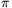/2] is concave below, from Jensen's inequality,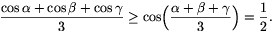It follows from the inequality of arithmetic and quadratic means, that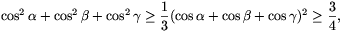which was the statement to prove. The solution also shows that equality only occurs in the case=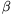=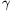=60o, that is, when the triangle O1O2O3 is equilateral, and so is the triangle ABC.

B. 3479. Find the minimum value of the expression 6t2+3s2-4st-8t+6s+5. (4 points)

Solution. The expression can be written in the form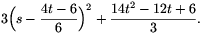With a fixed t, this is obviously a minimum when s=2t/3-1, and its minimum value is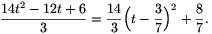This is a minimum for t=3/7. Thus the minimum of the expression is 8/7, which belongs to t=3/7.

B. 3480. Given are the points A, B, C and D in this order on the line e. What is the locus of those points P in the plane for which the angles APB and CPD are equal?  (5 points)

Gillis--Turán Mathematics Competition

Solution. On the line e, the points satisfying the condition are obviously those outside the closed segments AB and CD. Now let us only consider points that do not lie on the line. If the length of the segment AB equals the length of CD, then the points of the perpendicular bisector of segment AD are good, and it is easy to prove (e.g. with angles on the circumference of a circle) that no other points satisfy the condition. Assume henceforth that AB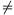CD. The equality of the angles APB and CPD is equivalent to the equality of the angles APC and BPD. Thus the points in question can be obtained as follows: For every 0<<, on both sides of the line e over the segments AC and BD, draw the arcs where the segments subtend angles of, and mark the intersection of the arcs.

We shall prove the following proposition: There exists exactly one circle such that the inversion with respect to that circle maps point A to D, and point B to C, and furthermore, the points in question are identical to the points of that circle (outside the line e). First suppose that k is such a circle. Its centre is obviously on the line e. Because of the angle-preserving property of inversion, the inversion with respect to k interchanges the arcs drawn over the segments AC and BD on each side of the line e. The intersections of the arcs are therefore mapped onto themselves, that is, the intersections all lie on the circle k. It is clear that all points of the circle k are obtained in this way, and it also follows from the reasoning that there can be only one such circle k, as the intersection of k with any particular arc corresponding to a certain angleis uniquely determined.

The circle k is obtained as follows: Construct the Thales circles over the segments AC and BD as diameters, and let P be one of their intersections. Let R denote the point of segment BC where the bisector of the angle BCP intersects the line e. Let O be the point on the line e for which the angles ORP and OPR are equal. With simple calculation we get that the angles OBP and OPC are also equal, and thus the triangle OBP is similar to triangle OPC. Therefore, OR2=OP2=OB.OC. The construction also shows that PR coincides with the bisector of angle APD, and hence OR2=OA.OD is also true. Thus the desired circle k is the circle of radius OR centred at O.

B. 3481. Let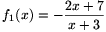, fn+1(x)=f1(fn(x)). Evaluate f2001(2002). (4 points)

Solution.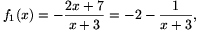therefore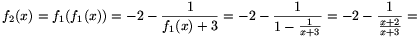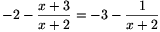and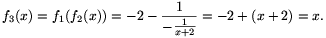And if f3k(x)=x for some positive integer k, then f3(k+1)(x)=f3(f3k(x))=f3(x)=x is also true. Hence with mathematical induction, f3k(x)=x, f2001(2002)=2002 for every positive integer k, as the calculation also shows that the functions fi are defined everywhere but at x=-2 and x=-3.